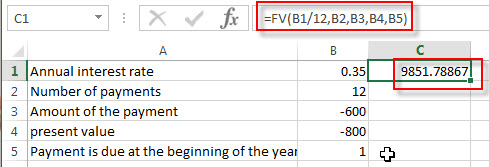# Excel FV Function

This post will guide you how to use Excel FV function with syntax and examples in Microsoft excel.

### Description

The Excel FV function used to calculate the future value of an investment based on a constant interest rate. So you can use the FV function to get the future value of an investment with either periodic, constant payments.

The FV function is a build-in function in Microsoft Excel and it is categorized as a Financial Function.

The FV function is available in Excel 2016, Excel 2013, Excel 2010, Excel 2007, Excel 2011 for Mac.

### Syntax

The syntax of the FV function is as below:

`=FV(rate,nper,pmt,[pv],[type])`

Where the FV function arguments are:

• Rate -This is a required argument. The interest rate per period.
• Nper– This is a required argument. The total number of payment periods for the annuity.
• Pmt– This is a required argument. The amount of the payment made each period. And if the Pmt argument is omitted, it will set as the default value 0.
• Pv – This is an optional argument. The present value of the payments. And if the PV argument is omitted, it will be set the default value as 0.
• Type – This is an optional argument. It indicates when the payments are due. And if the type argument is omitted, it will be set as 0.  And the Type argument can have two value 0 or 1.
 Set type equal to If payments are due 0 At the end of the period 1 At the beginning of the period

Note:

• The units for rate and nper should be consistent. If you make monthly payments on a four-year loan at 12 percent annual interest, and you should use 12%/12 for rate and 4*12 for nper. If you make annual payments on the same loan, you need to use 12% for rate and 4 for nper.
• For all the arguments, cash you pay out, such as deposits to savings, is represented by negative numbers; cash you receive, such as dividend checks, is represented by positive numbers.

### Excel FV Function Examples

The below examples will show you how to use Excel FV Function to calculate the future value of an investment with a constant interest rate.

#1 to get the future value of an investment, using the following formula:

`= FV (B1/12,B2,B3,B4,B5)`### Related Functions

• Excel IPMT Function
The Excel IPMT function used to calculate the interest payment for an investment based on a constant payment schedule and a constant interest rage.The syntax of the IPMT function is as below:= IPMT (rate, per, nper, pv, [fv], [type])…
• Excel IRR Function
The Excel IRR function returns the internal rate of return for a series of cash flows and the cash flows must be occurred at regular intervals (monthly or annually).The syntax of the IRR function is as below:=IRR(values, [guess])…
• Excel ISPMT Function
The Excel ISPMT function used to calculate the interest paid during a specific period of an investment.The syntax of the ISPMT function is as below:= ISPMT (rate, per, nper, pv)…
• Excel MIRR Function
The Excel MIRR function returns the modified internal rate of return for a series of cash flows and the cash flows must be occurred at regular intervals (monthly or annually). The syntax of the MIRR function is as below:=MIRR(values, finance_rate,reinvest_rate)…
Excel NPER Function
The Excel NPER function returns the number of periods for an investment or loan based on periodic payment amount and a constant interest rate.The syntax of the NPER function is as below:= NPER (rate, pmt, pv, [fv], [type])…
• Excel NPV Function
The Excel NPV function returns the net present value of an investment by using a discount rate and a series of future cash flows (future payments and income).The syntax of the NPV function is as below:= NPV (rate, Value1,[value2],…)…
• Excel PMT Function
The Excel PMT function returns the payment amount for a loan or investment based on constant payments and a constant interest rate.The syntax of the PMT function is as below:= PMT(rate, nper, pv,[fv],[type])…
• Excel PPMT Function
The Excel PPMT function returns the payment amount on the principal for a given period for a loan or investment based on constant payments and a constant interest rate. The syntax of the PPMT function is as below:=PPMT(rate, per,nper, pv,[fv],[type])…
• Excel RATE Function
The Excel RATE function returns the interest rate per payment period of an annuity.The syntax of the RATE function is as below:=RATE(nper, pmt,pv,[fv],[type],[guess])…
• Excel PV Function
The Excel PV function returns the present value of a loan or investment based on constant payments and a constant interest rate. So you can use the PV function to get the present value based on a series of future payments.The syntax of the PV function is as below:= PV(rate,nper,pmt,[fv],[type])…